Successfully reported this slideshow.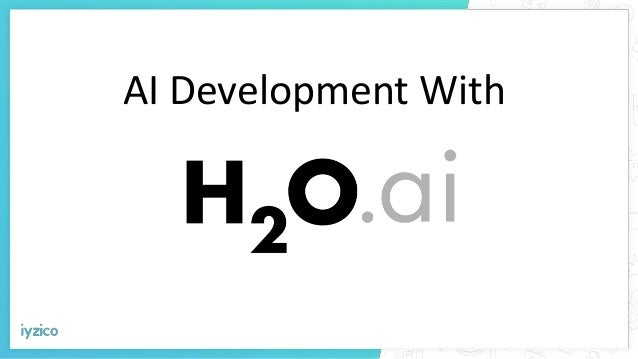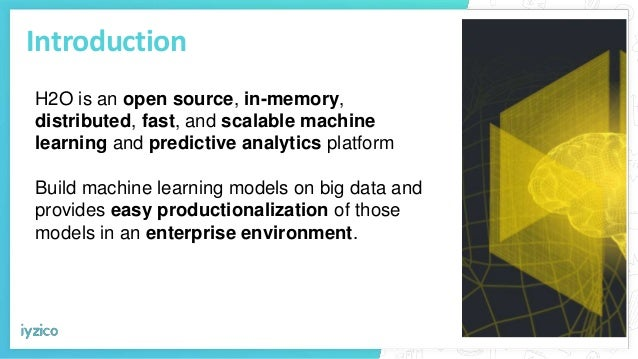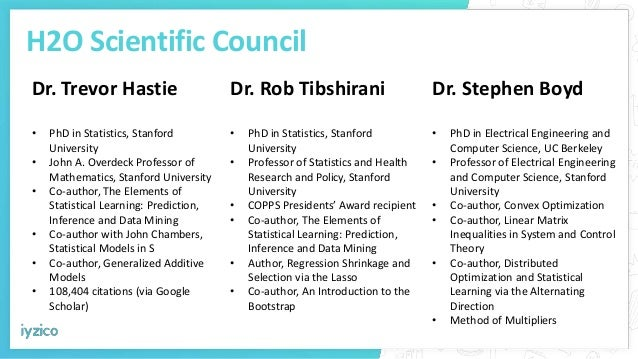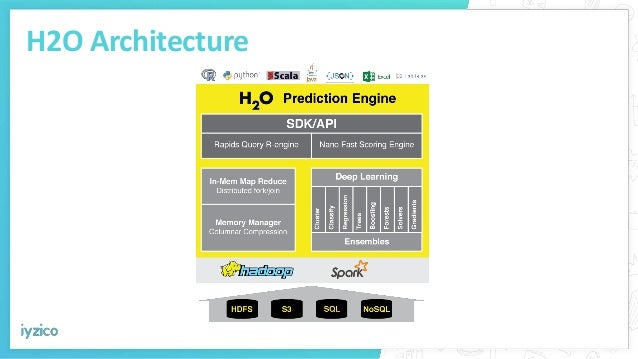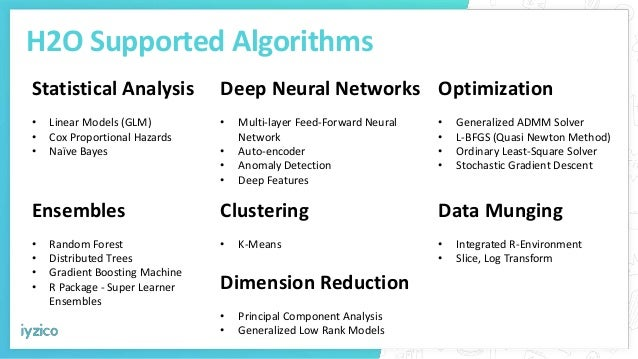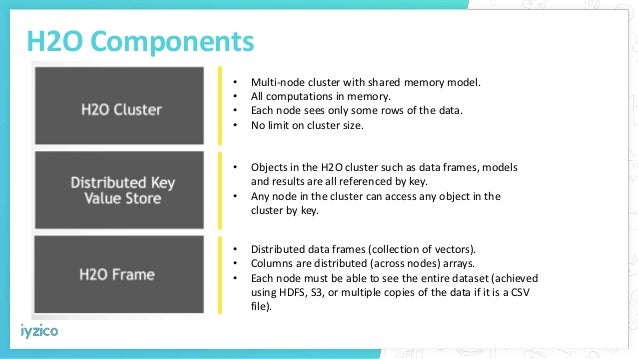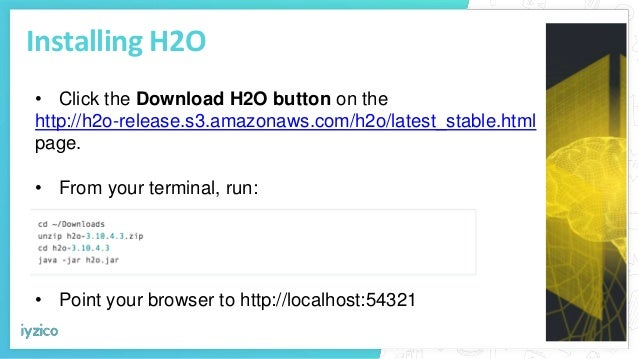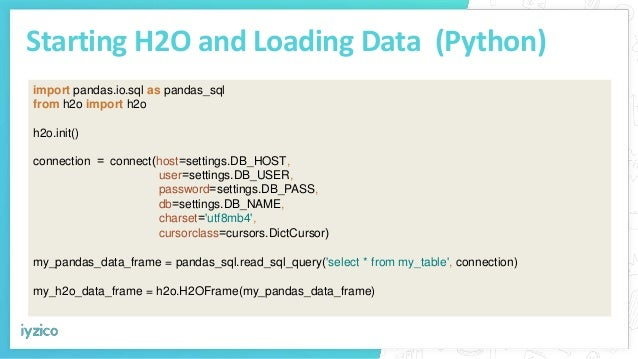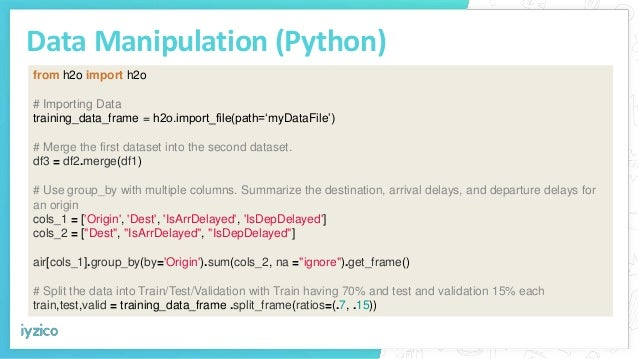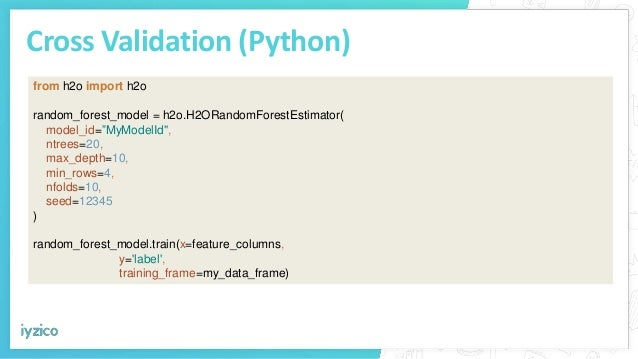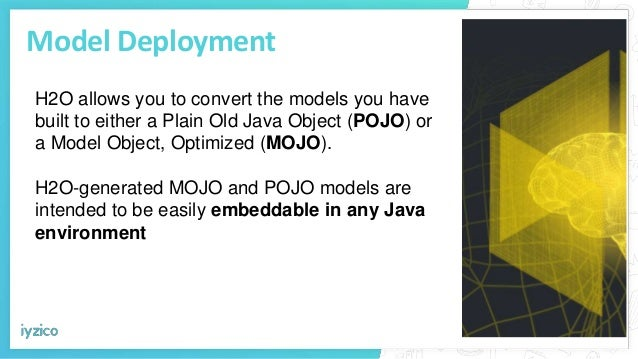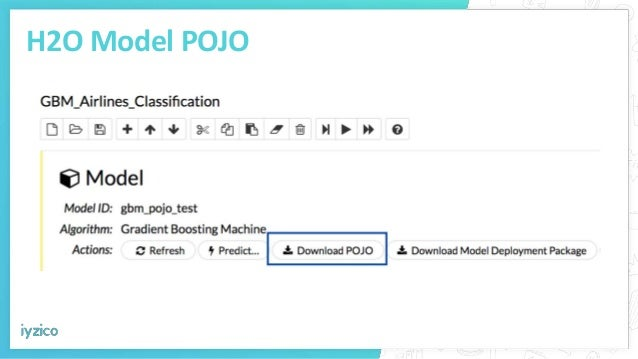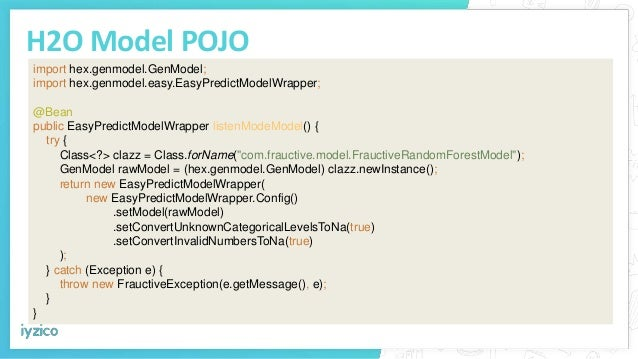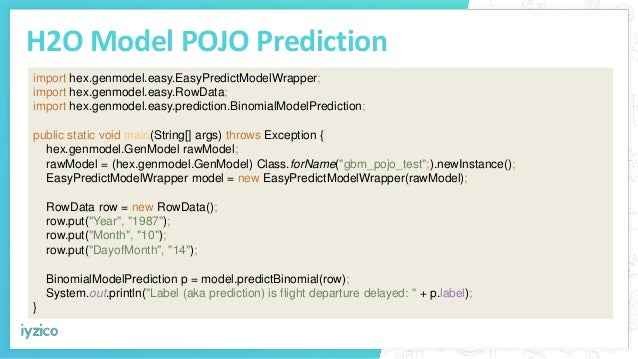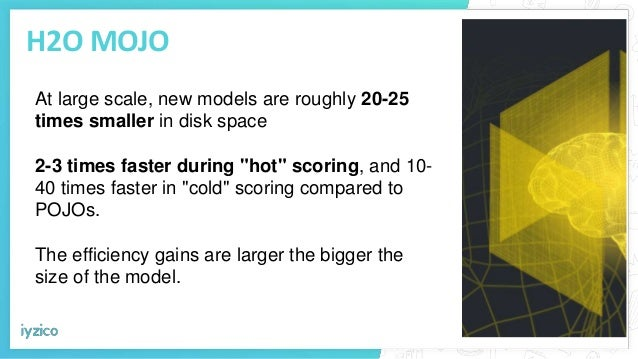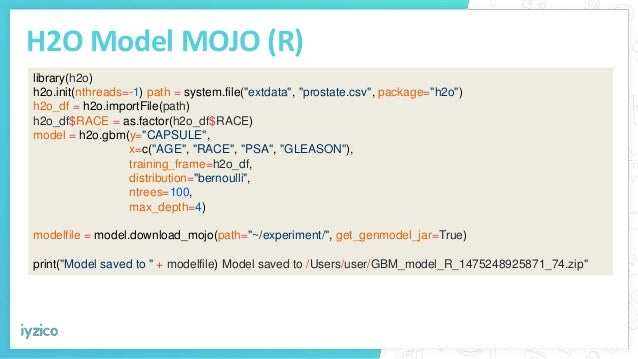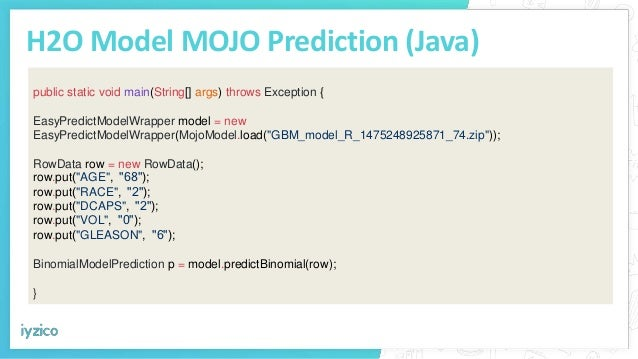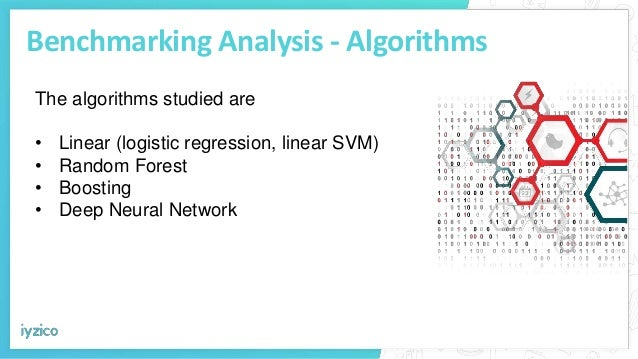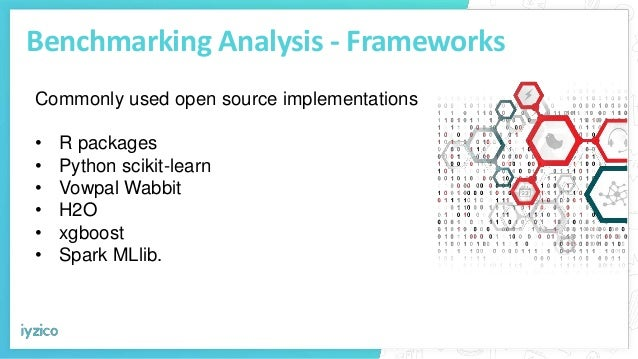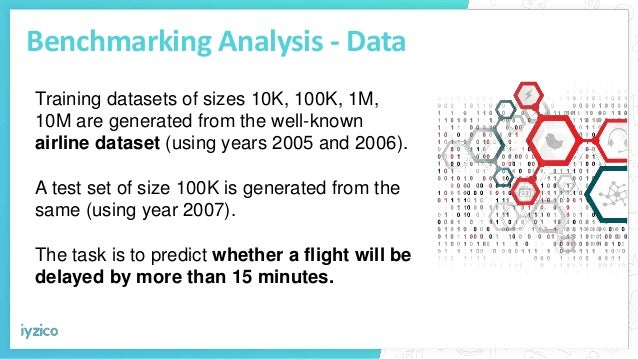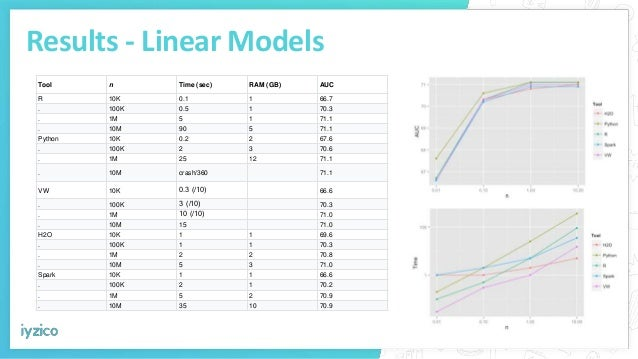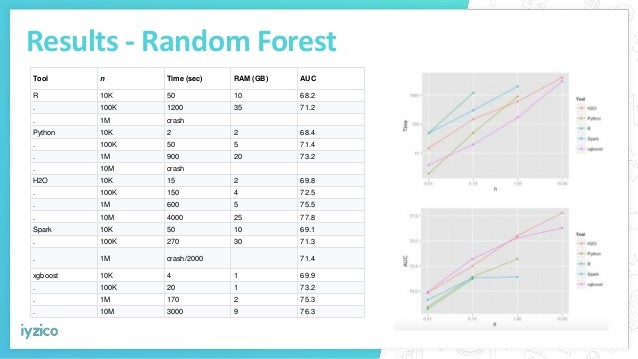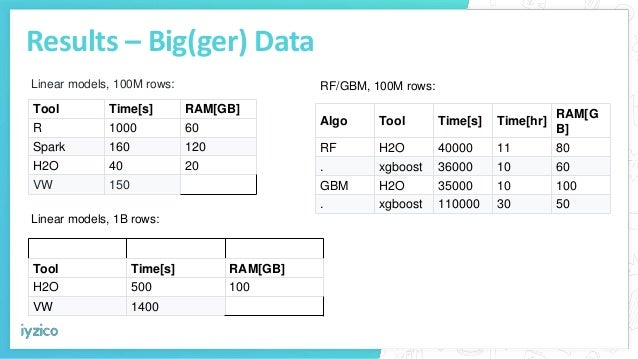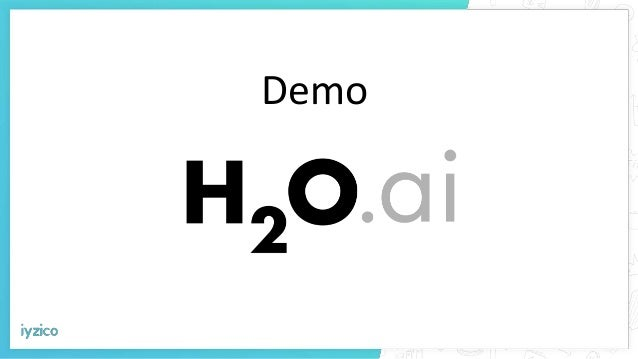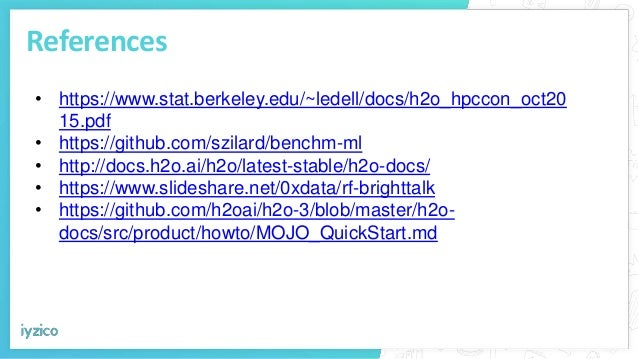Upcoming SlideShare
×

# AI Development with H2O.ai

H2O.ai basic components and model deployment pipeline presented. Benchmark for scalability, speed and accuracy of machine learning libraries for classification presented from https://github.com/szilard/benchm-ml.

• Full Name
Comment goes here.

Are you sure you want to Yes No• Be the first to comment

### AI Development with H2O.ai

1. 1. AI Development With
2. 2. Introduction H2O is an open source, in-memory, distributed, fast, and scalable machine learning and predictive analytics platform Build machine learning models on big data and provides easy productionalization of those models in an enterprise environment.
3. 3. H2O Scientific Council Dr. Trevor Hastie • PhD in Statistics, Stanford University • John A. Overdeck Professor of Mathematics, Stanford University • Co-author, The Elements of Statistical Learning: Prediction, Inference and Data Mining • Co-author with John Chambers, Statistical Models in S • Co-author, Generalized Additive Models • 108,404 citations (via Google Scholar) Dr. Rob Tibshirani • PhD in Statistics, Stanford University • Professor of Statistics and Health Research and Policy, Stanford University • COPPS Presidents’ Award recipient • Co-author, The Elements of Statistical Learning: Prediction, Inference and Data Mining • Author, Regression Shrinkage and Selection via the Lasso • Co-author, An Introduction to the Bootstrap Dr. Stephen Boyd • PhD in Electrical Engineering and Computer Science, UC Berkeley • Professor of Electrical Engineering and Computer Science, Stanford University • Co-author, Convex Optimization • Co-author, Linear Matrix Inequalities in System and Control Theory • Co-author, Distributed Optimization and Statistical Learning via the Alternating Direction • Method of Multipliers
4. 4. H2O Architecture
5. 5. H2O Supported Algorithms Statistical Analysis • Linear Models (GLM) • Cox Proportional Hazards • Naïve Bayes Ensembles • Random Forest • Distributed Trees • Gradient Boosting Machine • R Package - Super Learner Ensembles Deep Neural Networks • Multi-layer Feed-Forward Neural Network • Auto-encoder • Anomaly Detection • Deep Features Clustering • K-Means Dimension Reduction • Principal Component Analysis • Generalized Low Rank Models Optimization • Generalized ADMM Solver • L-BFGS (Quasi Newton Method) • Ordinary Least-Square Solver • Stochastic Gradient Descent Data Munging • Integrated R-Environment • Slice, Log Transform
6. 6. H2O Components • Multi-node cluster with shared memory model. • All computations in memory. • Each node sees only some rows of the data. • No limit on cluster size. • Objects in the H2O cluster such as data frames, models and results are all referenced by key. • Any node in the cluster can access any object in the cluster by key. • Distributed data frames (collection of vectors). • Columns are distributed (across nodes) arrays. • Each node must be able to see the entire dataset (achieved using HDFS, S3, or multiple copies of the data if it is a CSV file).
7. 7. Installing H2O • Click the Download H2O button on the http://h2o-release.s3.amazonaws.com/h2o/latest_stable.html page. • From your terminal, run: • Point your browser to http://localhost:54321
8. 8. Starting H2O and Loading Data (Python) import pandas.io.sql as pandas_sql from h2o import h2o h2o.init() connection = connect(host=settings.DB_HOST, user=settings.DB_USER, password=settings.DB_PASS, db=settings.DB_NAME, charset='utf8mb4', cursorclass=cursors.DictCursor) my_pandas_data_frame = pandas_sql.read_sql_query('select * from my_table', connection) my_h2o_data_frame = h2o.H2OFrame(my_pandas_data_frame)
9. 9. Data Manipulation (Python) from h2o import h2o # Importing Data training_data_frame = h2o.import_file(path=‘myDataFile’) # Merge the first dataset into the second dataset. df3 = df2.merge(df1) # Use group_by with multiple columns. Summarize the destination, arrival delays, and departure delays for an origin cols_1 = ['Origin', 'Dest', 'IsArrDelayed', 'IsDepDelayed'] cols_2 = ["Dest", "IsArrDelayed", "IsDepDelayed"] air[cols_1].group_by(by='Origin').sum(cols_2, na ="ignore").get_frame() # Split the data into Train/Test/Validation with Train having 70% and test and validation 15% each train,test,valid = training_data_frame .split_frame(ratios=(.7, .15))
10. 10. Cross Validation (Python) from h2o import h2o random_forest_model = h2o.H2ORandomForestEstimator( model_id=”MyModelId", ntrees=20, max_depth=10, min_rows=4, nfolds=10, seed=12345 ) random_forest_model.train(x=feature_columns, y='label', training_frame=my_data_frame)
11. 11. Model Deployment H2O allows you to convert the models you have built to either a Plain Old Java Object (POJO) or a Model Object, Optimized (MOJO). H2O-generated MOJO and POJO models are intended to be easily embeddable in any Java environment
12. 12. H2O Model POJO
13. 13. H2O Model POJO import hex.genmodel.GenModel; import hex.genmodel.easy.EasyPredictModelWrapper; @Bean public EasyPredictModelWrapper listenModeModel() { try { Class<?> clazz = Class.forName("com.frauctive.model.FrauctiveRandomForestModel"); GenModel rawModel = (hex.genmodel.GenModel) clazz.newInstance(); return new EasyPredictModelWrapper( new EasyPredictModelWrapper.Config() .setModel(rawModel) .setConvertUnknownCategoricalLevelsToNa(true) .setConvertInvalidNumbersToNa(true) ); } catch (Exception e) { throw new FrauctiveException(e.getMessage(), e); } }
14. 14. H2O Model POJO Prediction import hex.genmodel.easy.EasyPredictModelWrapper; import hex.genmodel.easy.RowData; import hex.genmodel.easy.prediction.BinomialModelPrediction; public static void main(String[] args) throws Exception { hex.genmodel.GenModel rawModel; rawModel = (hex.genmodel.GenModel) Class.forName("gbm_pojo_test";).newInstance(); EasyPredictModelWrapper model = new EasyPredictModelWrapper(rawModel); RowData row = new RowData(); row.put("Year", "1987"); row.put("Month", "10"); row.put("DayofMonth", "14"); BinomialModelPrediction p = model.predictBinomial(row); System.out.println("Label (aka prediction) is flight departure delayed: " + p.label); }
15. 15. H2O MOJO At large scale, new models are roughly 20-25 times smaller in disk space 2-3 times faster during "hot" scoring, and 10- 40 times faster in "cold" scoring compared to POJOs. The efficiency gains are larger the bigger the size of the model.
16. 16. H2O Model MOJO (R) library(h2o) h2o.init(nthreads=-1) path = system.file("extdata", "prostate.csv", package="h2o") h2o_df = h2o.importFile(path) h2o_df\$RACE = as.factor(h2o_df\$RACE) model = h2o.gbm(y="CAPSULE", x=c("AGE", "RACE", "PSA", "GLEASON"), training_frame=h2o_df, distribution="bernoulli", ntrees=100, max_depth=4) modelfile = model.download_mojo(path="~/experiment/", get_genmodel_jar=True) print("Model saved to " + modelfile) Model saved to /Users/user/GBM_model_R_1475248925871_74.zip"
17. 17. H2O Model MOJO Prediction (Java) public static void main(String[] args) throws Exception { EasyPredictModelWrapper model = new EasyPredictModelWrapper(MojoModel.load("GBM_model_R_1475248925871_74.zip")); RowData row = new RowData(); row.put("AGE", "68"); row.put("RACE", "2"); row.put("DCAPS", "2"); row.put("VOL", "0"); row.put("GLEASON", "6"); BinomialModelPrediction p = model.predictBinomial(row); }
18. 18. Benchmarking Analysis - Algorithms The algorithms studied are • Linear (logistic regression, linear SVM) • Random Forest • Boosting • Deep Neural Network
19. 19. Benchmarking Analysis - Frameworks Commonly used open source implementations • R packages • Python scikit-learn • Vowpal Wabbit • H2O • xgboost • Spark MLlib.
20. 20. Benchmarking Analysis - Data Training datasets of sizes 10K, 100K, 1M, 10M are generated from the well-known airline dataset (using years 2005 and 2006). A test set of size 100K is generated from the same (using year 2007). The task is to predict whether a flight will be delayed by more than 15 minutes.
21. 21. Results - Linear Models Tool n Time (sec) RAM (GB) AUC R 10K 0.1 1 66.7 . 100K 0.5 1 70.3 . 1M 5 1 71.1 . 10M 90 5 71.1 Python 10K 0.2 2 67.6 . 100K 2 3 70.6 . 1M 25 12 71.1 . 10M crash/360 71.1 VW 10K 0.3 (/10) 66.6 . 100K 3 (/10) 70.3 . 1M 10 (/10) 71.0 . 10M 15 71.0 H2O 10K 1 1 69.6 . 100K 1 1 70.3 . 1M 2 2 70.8 . 10M 5 3 71.0 Spark 10K 1 1 66.6 . 100K 2 1 70.2 . 1M 5 2 70.9 . 10M 35 10 70.9
22. 22. Results - Random Forest Tool n Time (sec) RAM (GB) AUC R 10K 50 10 68.2 . 100K 1200 35 71.2 . 1M crash Python 10K 2 2 68.4 . 100K 50 5 71.4 . 1M 900 20 73.2 . 10M crash H2O 10K 15 2 69.8 . 100K 150 4 72.5 . 1M 600 5 75.5 . 10M 4000 25 77.8 Spark 10K 50 10 69.1 . 100K 270 30 71.3 . 1M crash/2000 71.4 xgboost 10K 4 1 69.9 . 100K 20 1 73.2 . 1M 170 2 75.3 . 10M 3000 9 76.3
23. 23. Results – Big(ger) Data Tool Time[s] RAM[GB] R 1000 60 Spark 160 120 H2O 40 20 VW 150 Linear models, 100M rows: Tool Time[s] RAM[GB] H2O 500 100 VW 1400 Linear models, 1B rows: Algo Tool Time[s] Time[hr] RAM[G B] RF H2O 40000 11 80 . xgboost 36000 10 60 GBM H2O 35000 10 100 . xgboost 110000 30 50 RF/GBM, 100M rows:
24. 24. Demo
25. 25. References • https://www.stat.berkeley.edu/~ledell/docs/h2o_hpccon_oct20 15.pdf • https://github.com/szilard/benchm-ml • http://docs.h2o.ai/h2o/latest-stable/h2o-docs/ • https://www.slideshare.net/0xdata/rf-brighttalk • https://github.com/h2oai/h2o-3/blob/master/h2o- docs/src/product/howto/MOJO_QuickStart.md
26. 26. Thanks